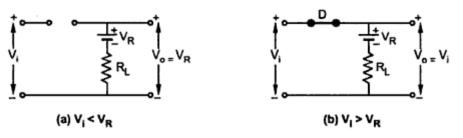### Clipping Below Reference Voltage VR

Series Clippers : Part5
By changing the orientation of the diode in the the circuit discussed previously, we get the clipping circuit which clips the portion of waveform, below the reference voltage VR.
The circuit is shown in the Fig. 1.Fig.  1 Clipping below VR

The diode D is an ideal diode which acts like a switch.
Operation :
When Vi is less than VR, the diode is reversed biased and circuit becomes open. The output voltage is equal to VR as no current flows in the circuit. The circuit is shown in the Fig. 2(a). When Vi is greater than VR, the diode becomes forward biased and circuit becomes as shown in the Fig. 2(b). The output voltage Vo is equal to the input voltage Vi.Fig. 2

The transfer characteristics equations are,
The transfer characteristics is shown in the Fig. 3.Fig. 3  Transfer characteristics

The region for Vi < VR is the clipping region while the region for Vi >VR is the transmitting region.
The Fig. 4(a) shows the sinusoidal input voltage Vi and the Fig. 4(b) shows the corresponding output waveform.Fig. 4 Clipping Below VR

Note : It can be seen that the portion of the output waveform below VR is clipped off.